Center of Mass Formula List for Different Shapes | JEE Main

Here is the center of mass formula list for different shapes. But, before we look at it, let’s examine the significance of center of mass.

So, center of mass of a group or system of particles is that (geometrical) point which behave as if

$\bullet$ all the mass of the system is located there &

$\bullet$ all the external forces are acting there

The above definition of center of mass is a result of the way, the position coordinates of center of mass of a group or system of particles is defined

$\overrightarrow{r}_{CM} = \cfrac{\sum_i m_i \overrightarrow{r}_i}{\sum_i m_i}$

Now, before we go any further, let’s quickly examine the difference between the terms center of mass and center of gravity.

For a rigid body, the gravitational force $\overrightarrow{F}_g$ effectively acts at a point, called the center of gravity. What this means is that, if the gravitational forces on the individual particles of the rigid body were somehow turned OFF and force $\overrightarrow{F}_g$ were to be turned ON at center of gravity, the net force and net torque (about any point) on the rigid body due to gravitational pull would not change and if the gravitational acceleration $g$ is same for all particles of the body (which will be true for any rigid body that we would encounter in our day to day lives), then the center of mass and center of gravity would coincide. If this didn’t make sense and you would like to understand more, sign up for free and go through the lecture video ‘Center of mass vs center of gravity’

With that, let’s review the center of mass of a two particle system, of a system of three particles, of a group of simple rigid bodies (for example a uniform $I$ shaped lamina), of a non-uniform rod of length $l$, of an arc, of a uniform circular arc, of a semicircular ring, of a semicircular disc, of an annular semicircular disc, of a triangle, of a solid hemisphere, of a hollow hemisphere, of a hollow cone, of a solid cone

Center of mass of a two particle system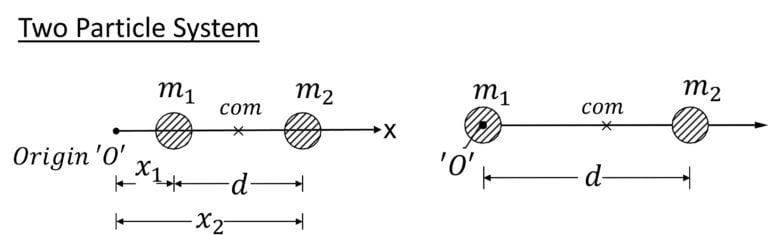For the scenario on the left:
$x_{CM} = \cfrac{m_1 x_1 + m_2 x_2}{m_1 + m_2}$ $=x_1 + \cfrac{m_2 d}{m_1 + m_2}$

For the scenario on the right
$x_{CM} = \cfrac{m_2 d}{m_1 + m_2}$

Note:
(i) If $m_1 > m_2$, $x_{CM} < \cfrac{d}{2}$ (i.e. x_{CM} is located closer to $m_1$)
(ii) If
$m_1 < m_2$, $x_{CM} > \cfrac{d}{2}$ (i.e. x_{CM} is located closer to $m_2$)

Center of mass of a system of multiple particles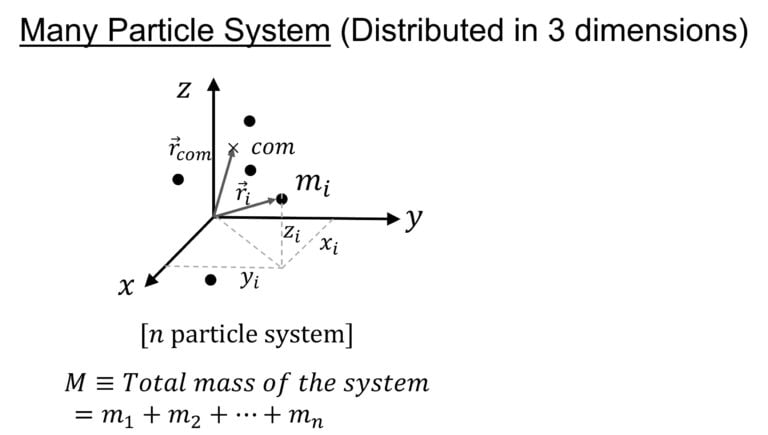$x_{CM} =$ $\cfrac{1}{M} \sum_{i=1}^n m_i x_i$
$y_{CM} =$ $\cfrac{1}{M} \sum_{i=1}^n m_i y_i$
$z_{CM} =$ $\cfrac{1}{M} \sum_{i=1}^n m_i z_i$
So, position vector of center of mass can be written as
$\overrightarrow{r}_{CM}=$ $x_{CM} \hat{i} +$ $y_{CM} \hat{j} +$ $z_{CM} \hat{k}$
Or, $\overrightarrow{r}_{CM} =$ $\cfrac{1}{M} \sum_{i=1}^n m_i \overrightarrow{r}_i$
where $\overrightarrow{r}_i=$ $x_i \hat{i} +$ $y_i \hat{j} +$ $z_i \hat{k}$

Center of mass of a group of simple rigid bodies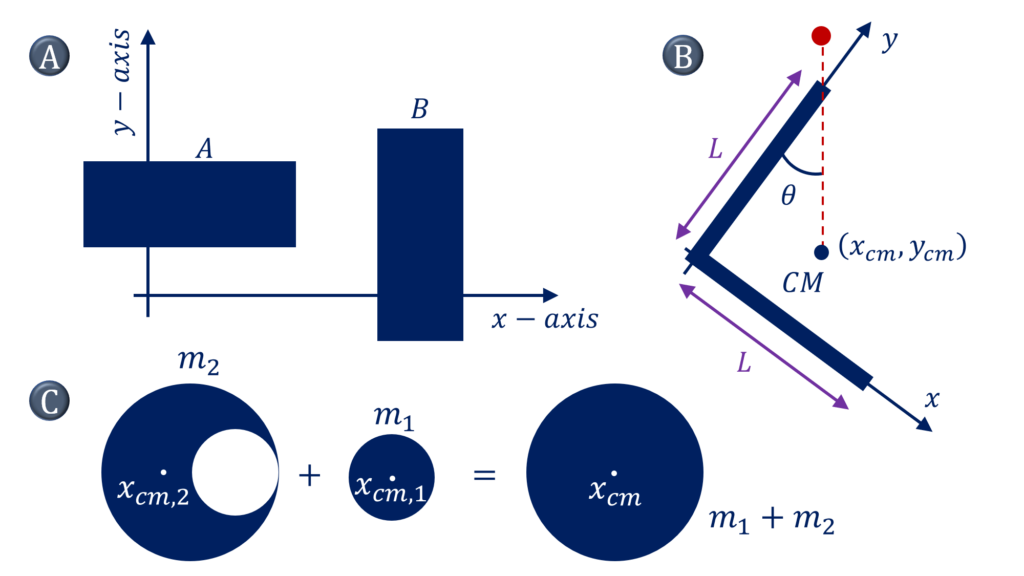For a system comprising of two or more simple rigid bodies (with the known center of masses), let’s determine the center of mass of the system. OR for a simple rigid body, such as a disc, with a cut out of a standard shape (for example circle or square, etc.), let’s determine its center of mass

$\color{blue}{\text{Solution Steps}}$
A : $x_{cm} =$ $\cfrac{\sum m_i x_i}{\sum m_i}$
$=\cfrac{\sum_A m_ix_i + \sum_B m_ix_i}{m_A+m_B}$
$= \cfrac{m_Ax_{cm,A} + \sum_B m_B x_{cm,B}}{m_A+m_B}$
$y_{cm} = \cfrac{\sum m_i y_i}{\sum m_i}$
$=\cfrac{\sum_A m_iy_i + \sum_B m_iy_i}{m_A+m_B}$
$= \cfrac{m_Ay_{cm,A} + \sum_B m_B y_{cm,B}}{m_A+m_B}$

B) : $\tan \theta = \cfrac{x_{cm}}{L-y_{cm}}$
$x_{cm} = \cfrac{m.0+m.L/2}{2m}, \quad y_{cm}$
$= \cfrac{m.L/2 + m.0}{2m}$
$x_{cm} = y_{cm} = \cfrac{L}{4}$
$\theta = \tan^{-1} \cfrac{1}{3}$

C) : $(m_1 + m_2)x_{cm}$ $= m_1x_{cm,1} + m_2 x_{cm,2}$
$x_{cm} = 0$
$x_{cm,2} = – \cfrac{m_1x_{cm,1}}{m_2}$

Center of mass of a uniform $I$ shaped lamina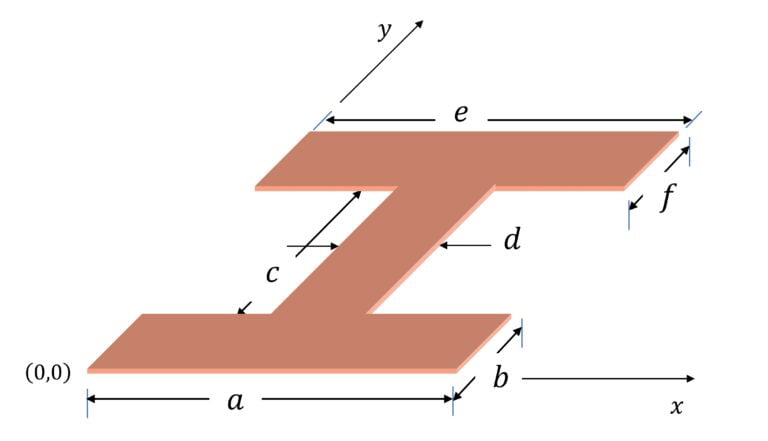Center of mass of $I$ shaped lamina (of uniform surface density $\sigma$):

$x_{CM} = \cfrac{ab\cfrac{a}{2}+cd\cfrac{a}{2}+ef\cfrac{a}{2}}{ab+cd+ef}$

$y_{CM} = \cfrac{ab\cfrac{b}{2}+cd\cfrac{2b+c}{2}+ef\cfrac{2b+2c+f}{2}}{ab+cd+ef}$

Center of mass of a non uniform rod of length $l$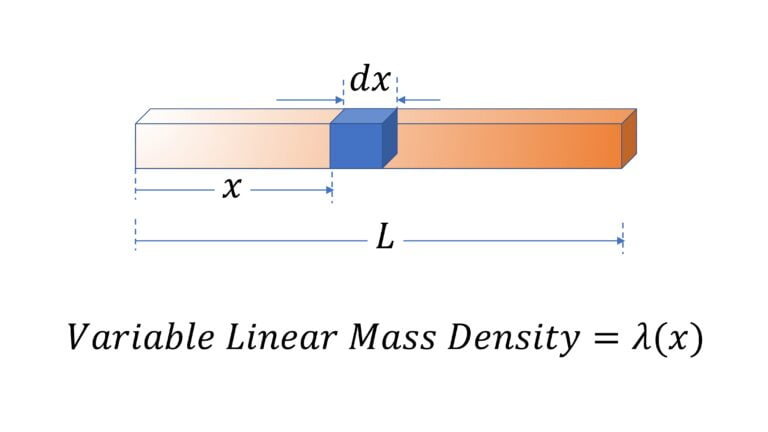$x_{cm} =$ $=\cfrac{\int_0^L x \lambda(x) dx}{\int_0^L \lambda(x) dx}$

$x_{cm} =$ $\cfrac{\int x dm}{\int dm}$ $=\cfrac{\int_0^L x \lambda(x) dx}{\int_0^L \lambda(x) dx}$

Center of mass of arc (of a ring)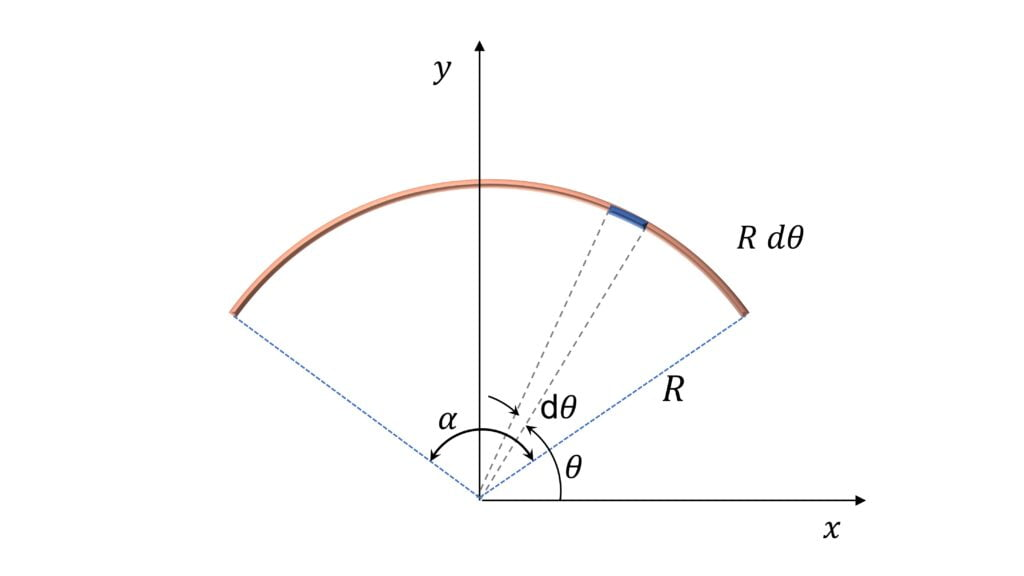$x_{cm} = 0$
$y_{cm}=\cfrac{R \sin (\alpha/2)}{(\alpha / 2)}$

$x_{cm} = 0$ because of symmetry of the arc about the $y-$axis

$y_{cm} = \cfrac{\int y dm}{\int dm}$
$=\cfrac{R^2 \int_{\cfrac{\pi -\alpha}{2}}^{\cfrac{\pi + \alpha}{2}} \sin \theta}{R \int_{\cfrac{\pi -\alpha}{2}}^{\cfrac{\pi + \alpha}{2}} \theta}$
$=\cfrac{R \sin (\alpha/2)}{(\alpha / 2)}$

Center of mass of uniform circular arc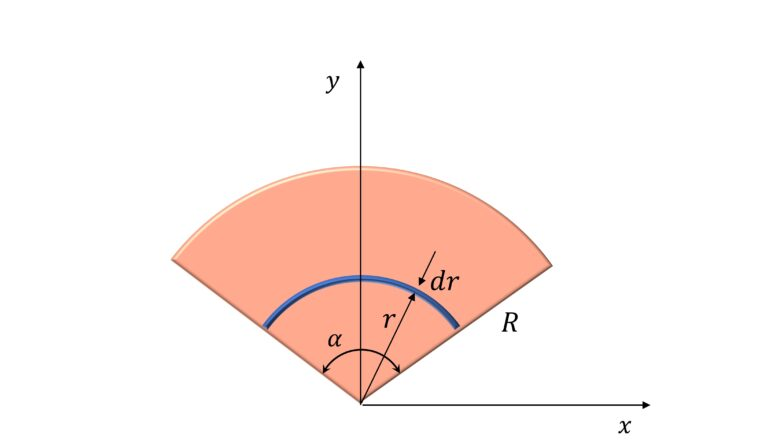$x_{cm} = 0$ $y_{cm} =$ $\cfrac{2}{3} R \cfrac{ \sin ( \alpha / 2)}{( \alpha / 2)}$

$x_{cm} = 0$ because of symmetry about the $y-$axis

$y_{cm} = \cfrac{\int ydm}{\int dm}$

$dm = \sigma r \alpha dr$

$y_{cm} = \cfrac{\int_0^R r \cfrac{\sin (\alpha /2)}{\alpha /2} r \alpha dr}{\int_0^R r \alpha dr}$

$=\cfrac{2}{3} R \cfrac{\sin (\alpha /2)}{(\alpha /2)}$

Center of mass of semicircular ring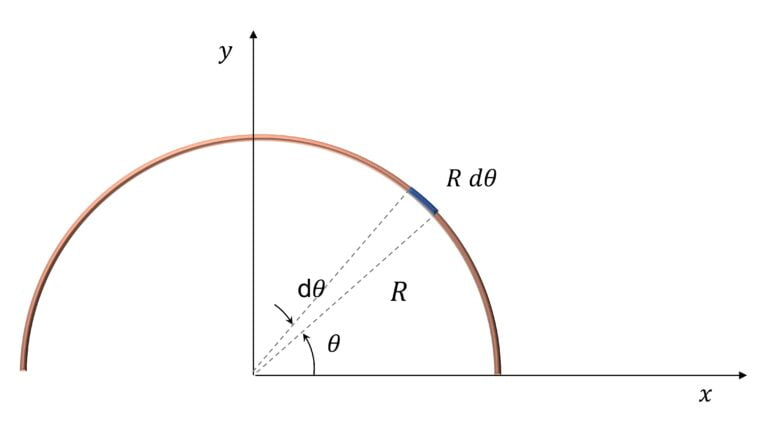Coordinates of center of mass of semicircular ring are:
$x_{cm} = 0$
$y_{cm} = \cfrac{R \sin (\pi / 2)}{(\pi / 2)}$ $=\cfrac{2R}{\pi}$ (Refer the center of mass of arc)

Center of mass of semicircular disc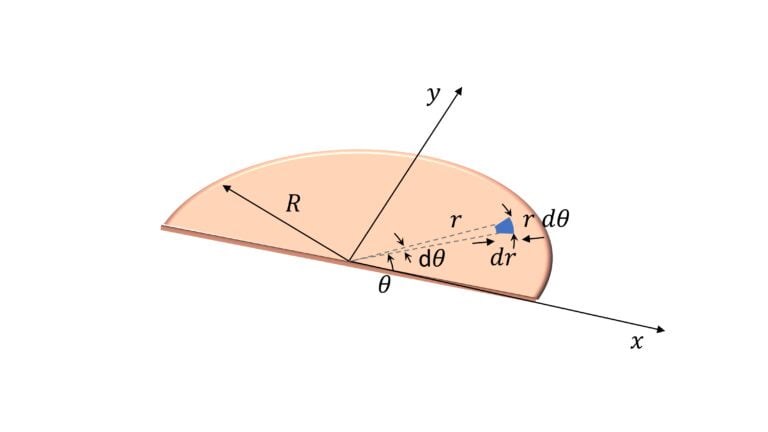$x_{cm} = 0$
$y_{cm} = \cfrac{4R}{3 \pi}$

Approach 1: Use the expression derived for uniform circular arc (disc), where we found that $y_{cm} = \cfrac{2}{3} R \cfrac{\sin (\alpha /2)}{(\alpha /2)}$

Approach 2:
$y_{cm} = \cfrac{\int ydm}{\int dm}$
$y = r \sin \theta$
$dm = \sigma r dr d \theta$

$\implies$ $y_{cm} = \cfrac{\sigma \int_0^R r^2 dr \int_0^{\pi} \sin \theta d \theta}{\sigma \int_0^R r dr \int_0^{\pi} d \theta}$
$=\cfrac{4R}{3 \pi}$

Center of mass of annular semicircular disc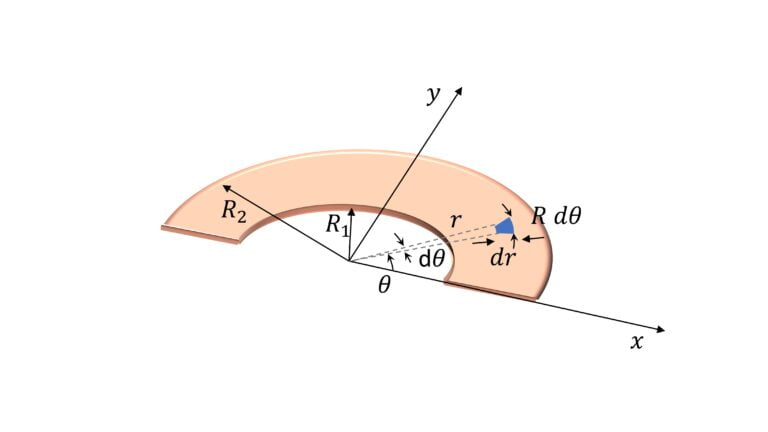$x_{cm}=0$
$y_{cm} = \cfrac{4}{3 \pi} \cfrac{R_1^2 + R_1 R_2 + R_2^2}{R_1+R_2}$

$y_{cm} = \cfrac{\int ydm}{\int dm}$
$y = r \sin \theta$
$dm = \sigma r dr d \theta$

$\implies$ $y_{cm} = \cfrac{\sigma \int_0^R r^2 dr \int_0^{\pi} \sin \theta d \theta}{\sigma \int_0^R r dr \int_0^{\pi} d \theta}$
$=\cfrac{4}{3 \pi} \cfrac{R_1^2 + R_1 R_2 + R_2^2}{R_1+R_2}$

Center of mass of a triangle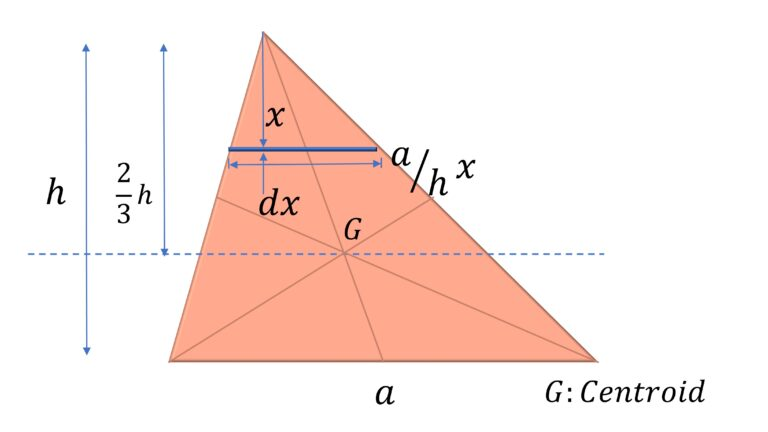$x_{cm} = \cfrac{2}{3}h$

$x_{cm} = \cfrac{xdm}{dm}$
$dm = \cfrac{a}{h} x dx$
$x_{cm} = \cfrac{(a/h) \int_0^h x^2dx}{(a/h) \int_0^h xdx}$

Center of mass of solid hemisphere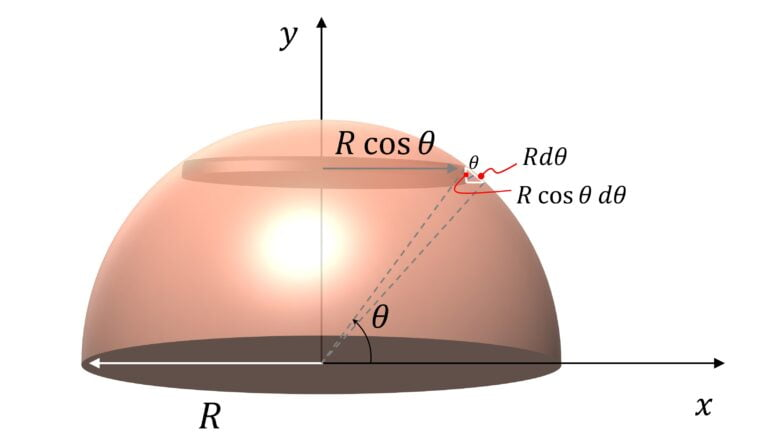$x_{cm} = 0$
$y_{cm} = \cfrac{3R}{8}$

$x_{cm} = 0$ because of symmetry about $y-$axis

$y_{cm} = \cfrac{\int ydm}{\int dm}$

$dm = \pi (R \cos \theta)^2 (R \cos \theta) d \theta$ $=\pi R^3 \cos^3 \theta d \theta$
$y = R \sin \theta$
So,
$y_{cm} = R\cfrac{\int_0^{\pi/2} \sin \theta \cos^3 \theta d \theta}{\int_0^{\pi/2} \cos^3 \theta d \theta}$

Solving we get $y_{cm} = \cfrac{3R}{8}$

Center of mass of hollow hemisphere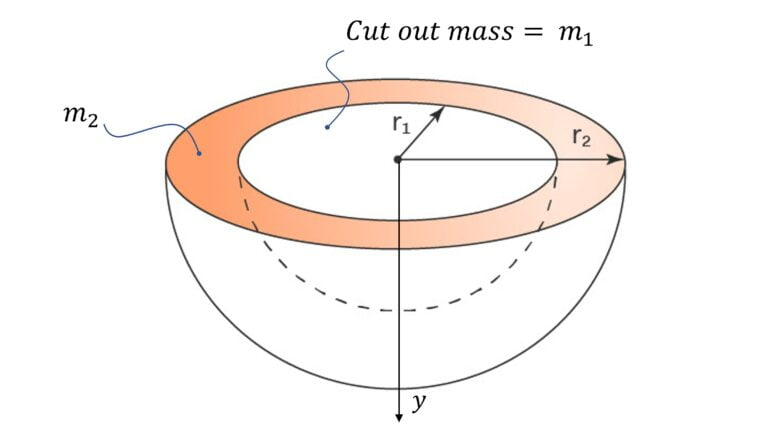$y_{cm} = \cfrac{3}{8} \cfrac{(R_2^2 + R_1^2)(R_1 + R_2)}{R_2^2 +R_1 R_2 + R_1^2}$

$(m_1 + m_2) \cfrac{3R_2}{8} = m_2 y_{cm} + m_1 \cfrac{3R_1}{8}$

$m_1 = \cfrac{4}{3} \pi R_1^3 \rho$

$m_2 = \cfrac{4}{3} \pi (R_2^3 – R_1^3) \rho$

Solving we get
$y_{cm} = \cfrac{3}{8} \cfrac{(R_2^2 + R_1^2)(R_1 + R_2)}{R_2^2 +R_1 R_2 + R_1^2}$

Center of mass of solid cone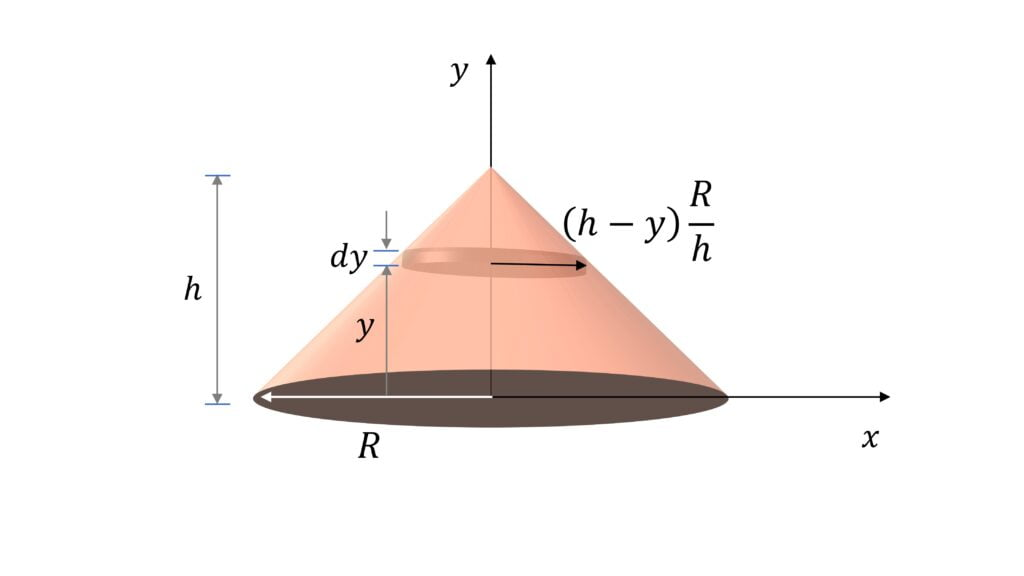$x_{cm} = 0$
$y_{cm} = \cfrac{h}{4}$

$y_{cm} = \cfrac{\int y dm}{\int dm}$

$dm = \pi (h-y)^2 \cfrac{R^2}{h^2} dy \rho$

$\int dm = \cfrac{1}{3} \pi R^2 h \rho$

Solving we get
$y_{cm} = \cfrac{h}{4}$

Center of mass of hollow cone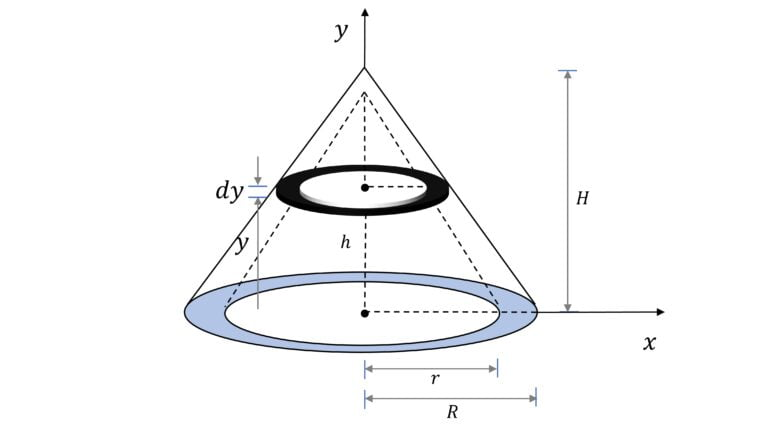As measured from the bottom, $y_{cm} = \cfrac{1}{4} \cfrac{R^2 H^2 – r^2 h^2}{R^2H – r^2 h}$

$(m+M) \cfrac{H}{4} = m \cfrac{h}{4} + M y_{cm}$

$m=\cfrac{1}{3}\pi r^2 h \rho$

$M = \cfrac{1}{3} \pi \rho (R^2H – r^2 h)$

Solving we get
$y_{cm} = \cfrac{1}{4} \cfrac{R^2 H^2 – r^2 h^2}{R^2H – r^2 h}$